### Create an Account

Already have account?

### Forgot Your Password ?

Home / Questions / For the circuit shown in Figure P1310 a Determine the reluctance values and show the...

# For the circuit shown in Figure P1310 a Determine the reluctance values and show the magnetic circuit assuming that

For the circuit shown in Figure P13.10:

a.    Determine the reluctance values and show the magnetic circuit, assuming that μ = 3,000 μ0.

b.    Determine the inductance of the device.

c.    The inductance of the device can be modified by cutting an air gap in the magnetic structure. If a gap of 0.1 mm is cut in the arm of length l3, what is the new value of inductance?

d.    As the gap is increased in size (length), what is the limiting value of inductance? Neglect leakage flux and fringing effects.

1 cm

2 cm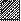Cross section 1 cm

1 cm

Figure P13.11

1cm 1 cm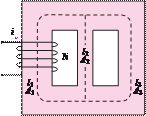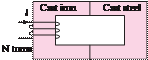Figure P13.12

13.13 Find the magnetic flux φ established in the series magnetic circuit of Figure P13.13.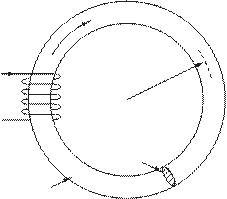f

= 100 turns

l1 = 30 cm

A1 = 100 cm2

l2 = 10 cm

A2 = 25 cm2

l3 = 30 cm

A3 = 100 cm2

= 2 A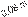= 100 turns

F

Jun 18 2020 View more View Less

#### Answer (Solved)Subscribe To Get Solution